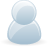# Support Forum

or## Reading Voltage to Determine Resistance

1767 Views - Created 08/05/2018

08/05/2018

Posted by:
vvarriorI currently have a project where I need to determine the value of a resistor in a voltage divider circuit.The formula I am using is the following:

r2 = (-v1*r1)/(v1-v2)

When I check the values of the voltage of this circuit using 18 bit, this is my result using 330 ohm resistor on r1:

v1 v2 r1 r2 r2 (calculated)
3.175119 3.299596 330 15,000 8417.533119

For the purpose of this test, I do know the value of r2 (15,000 ohms) but in application I won't know. I see the schematic for ADCPi plus shows 10k, vout, 6.8k on the voltage divider, but how I would verify the resistance of the board?

My feeling is that r2 is being affected by the input resistance in the voltage divider on the ADCPi plus board. Can you give any insight into the problem I am having?

08/05/2018

Posted by:
andrewLocation:
United Kingdom

The problems you are having are most likely being caused by the voltage divider and the inputs are single-ended so they are being measured relative to ground. Ideally, you need a differential ADC input which measures the voltage difference between two points. That way you can measure each side of the resistor and read the voltage across the resistor.

The MCP3424 ADC chips used on the ADC Pi Plus are designed to be differential or single-ended inputs but on the ADC Pi Plus, they are configured as single-ended inputs. We do sell a differential version of the ADC Pi Plus called the ADC Differential Pi. It is possible to convert the ADC Pi Plus to differential inputs but it involves removing the input resistors and drilling the PCB.

This forum post details someone else who had a similar problem to you and I gave a description of how to modify the ADC Pi Plus to make the inputs differential.

The differential inputs on the MCP3424 ADC are only able to read +-2.048V so if the voltage across your resistor is going to exceed that limit you will need to use an operational amplifier to reduce the voltage down to a level below 2.048V.

08/05/2018

Posted by:
vvarriorI did read that other post you linked to me. I can try drilling out the board but isn't there a formula to account for the additional resistance? Perhaps a way to find out the resistance and add that into my formula?

If drilling out the board works, I will plan on using the differential pis on top of pi zeros.

09/05/2018

Posted by:
vvarriorIn fact, I was able to do exactly that... Accounting for the resistance using the following formula:

k = 16800
r2 = ((r1*Vout)*(k)) / (((r1*Vout)/(Vin-Vout))-(k))